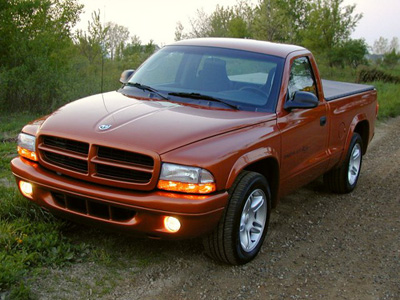# Dodge Dakota R/T Information

###### 2001 Dodge Dakota R/T Information:Above: 2001 Dodge Dakota R/T by Fred Schramm . Featured June 2001 in the Mopars Of The Month site. Click Here to check it out.

Standing out from the compact pickup crowd is the Dakota R/T. This limited-edition performance pickup is equipped with a 5.9-liter Magnum V-8 engine and a large-bore, free-flow exhaust that delivers increased engine performance.

A powerful exhaust note backs up the truck’s performance capability. The 250 horsepower engine is mated to a four-speed transmission, and is supported by massive 255/55×17-inch tires. The Dakota R/T is lowered one inch and features unique suspension tuning and front and rear stabilizer bars for added control. Further enhancing its street truck reputation, Dakota R/T is only offered in two-wheel drive.

For 2001, all Dakota models get a fresh new interior and a host of new customer convenience features that widen the gap from the competition. Atlantic Blue, Bright Silver and Graphite Metallic were added to color choices.

###### 2001 Dodge Dakota R/T Production Numbers

Regular Cab Color:

• Atlantic Blue: (USA) = 5 – (Canada) = 0 – (Export) = 0 – Total = 5
• Amber Fire: (USA) = 12 – (Canada) = 1 – (Export) = 0 – Total = 13
• Black: (USA) = 186 – (Canada) = 15 – (Export) = 3 – Total = 204
• Bright Silver: (USA) = 143 – (Canada) = 11 – (Export) = 4 – Total = 158
• Bright White: (USA) = 43 – (Canada) = 2- (Export) = 1 – Total = 46
• Flame Red: (USA) = 116 – (Canada) = 3 – (Export) = 6 – Total = 125
• Graphite Metallic: (USA) = 28 – (Canada) = 4 – (Export) = 1 – Total = 33
• Intense Blue: (USA) = 32 – (Canada) = 2 – (Export) = 0 – Total = 34

Total 2001 Dakota R/T Regular Cab Production = 618

Club Cab Color:

• Atlantic Blue: (USA) = 16 – (Canada) = 0 – (Export) = 0 – Total = 16
• Amber Fire: (USA) = 35 – (Canada) = 2 – (Export) = 0 – Total = 37
• Black: (USA) = 476 – (Canada) = 62 – (Export) = 0 – Total = 538
• Bright Silver: (USA) = 538 – (Canada) = 77 – (Export) = 0 – Total = 615
• Bright White: (USA) = 144 – (Canada) = 7- (Export) = 0 – Total = 151
• Flame Red: (USA) = 312 – (Canada) = 70 – (Export) = 1 – Total = 383
• Graphite Metallic: (USA) = 67 – (Canada) = 8 – (Export) = 0- Total = 75
• Intense Blue: (USA) = 113 – (Canada) = 19 – (Export) = 1 – Total = 133

Total 2001 Dakota R/T Club Cab Production = 1,948